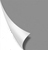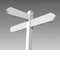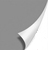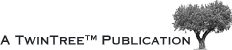##A|B|C|D|E|F|G|H|I|J|K|L|M|N
O|P|Q|R|S|T|U|V|W|X|Y|Z|α-ω

## Glossary • X

x: dimension in the stationary (laboratory) frame of reference in the plane orthogonal (at right angles) to the direction of the static magnetic field (B0), z and orthogonal to y.

x': dimension in the rotating frame of reference in the plane orthogonal to the direction of the static magnetic field (B0), z.

## Glossary • Y

Vector: a quantity having both magnitude and direction, frequently represented by an arrow whose length is proportional to the magnitude. y: dimension in the stationary (laboratory) frame of reference in the plane orthogonal to the direction of the static magnetic field (B0), z, and orthogonal to the other dimension in this plane, x.

y': dimension in the rotating frame of reference in the plane orthogonal to the direction of the static magnetic field (B0), z, and orthogonal to the other dimension in this plane, x'.

## Glossary • Z

z: dimension in the direction of the static magnetic field (B0 and H0), in both the sta­tio­nary and rotating frames of reference.

Zeugmatography: original term for magnetic resonance imaging (MRI) coined by Paul C. Lauterbur suggesting the role of the gradient magnetic field in joining the RF magnetic field to a de­sired local spatial region through nuclear magnetic resonance (NMR).

Missing terms? Send us an e-mail. We'll add them ...